# Vertex-edge graphs part 1

Title: How to represent real life examples in Vertex-edge graphs.
Subject Area: Algebra 1: Vertex-edge graphs
Grade Level: Three different classes consisting of 9th or 10th, 11th and 12th graders. First exposure to vertex-edge graphs.
Length: 40 minute class
National standards: Principles and Standards for School Mathematics, National Council of Teachers of Mathematics (NCTM), 2000.

Wholeness of the lesson Vertex-edge graphs consists of dots and lines that allow us to represent and solve real-life problems.

Objectives After this lesson you will understand that …

• Vertex is a node or point where lines can meet. Vertex can have degree 0 to n, depending on how many edges are connected.
• Edge means a line that connects two vertices.
• Walk describes a path traveled using edges and vertices.
• Vertices and Edges can represent real-life situations and problems, helping us to solve them.
• Euler path or cycle is a trail in a graph which visits every edge exactly once
• Hamiltonian path or cycle is a trail in a graph which visits every vertex exactly once

After this lesson you will be able to…

• Draw vertex-edge graphs
• Represent a real-life example as vertex-edge graph
• Solve a problem using vertex-edge graph
• Determine whether a trail is Hamiltonian or Euler path / cycle

After this lesson you will appreciate…

• The logic, beauty and practicality of graph theory of Mathematics.

Approach Direct lesson with indirect components combined with group activity and collaborative learning method.

Main Points

1. Vertex is a point or a node where lines can meet.
2. Degree of a vertex equals to number of lines meeting at the vertex.
3. Edge is a line that connects two vertices.
4. Walk is a path traveled using edges and vertices.
5. If edges have a direction, we call it a digraph.
6. Graph means a set of finite number of vertices connected by edges.
7. Graphs, consisting of vertices and edges can represent real-life situations and problems, helping us solve them.
8. If the graph starts and ends in the same vertex, it’s called a circuit or cycle.
9. If each edge is visited only once, it’s an Euler path or cycle.
10. If each vertex is visited only once, it’s a Hamiltonian path or cycle.

Lesson Procedures

1. Launch So far we have been looking at exponents and rules that they follow. Today we are going to shift gears and go into something completely different… We are going to look at one of the geometric diagrams called vertex-edge graphs. This is a basis for more problem solving in Algebra and Geometry.

Attention Step and Motivation Step

Example 1: The Lunch break is pretty short, so you will need to know where to go during this time.

While Mrs. Myers hands out yesterday’s homework, design a path on this map for your lunch break:

Let’s see an example path you might drive (or your friend would drive) over the lunch break:

View Larger Map

See how all the stops are vertices and the road=path=walk (technical term) connecting the vertices are edges. The Google Map shows the optimal route

• starting with the Fairfield High School, going through
• Fairfield Public Library to return books,
• Subway for quick lunch,
• Co-Ed movie theater to see what to do in the evening,
• and get back to class before the next class.
All of the above are milestones or stops on the way. We say that they are vertices. The paths between vertices  called edges.
Note that there are many ways to connect the vertices. The order and the relationships between the vertices are what matter.
Also note that this graph is a digraph, because there is a direction to each of the edge.
In the next example we will see even more elaborate vertex-edge graph.

Example 2: Take the first London Underground (Tube i.e. Metro) map from 1933: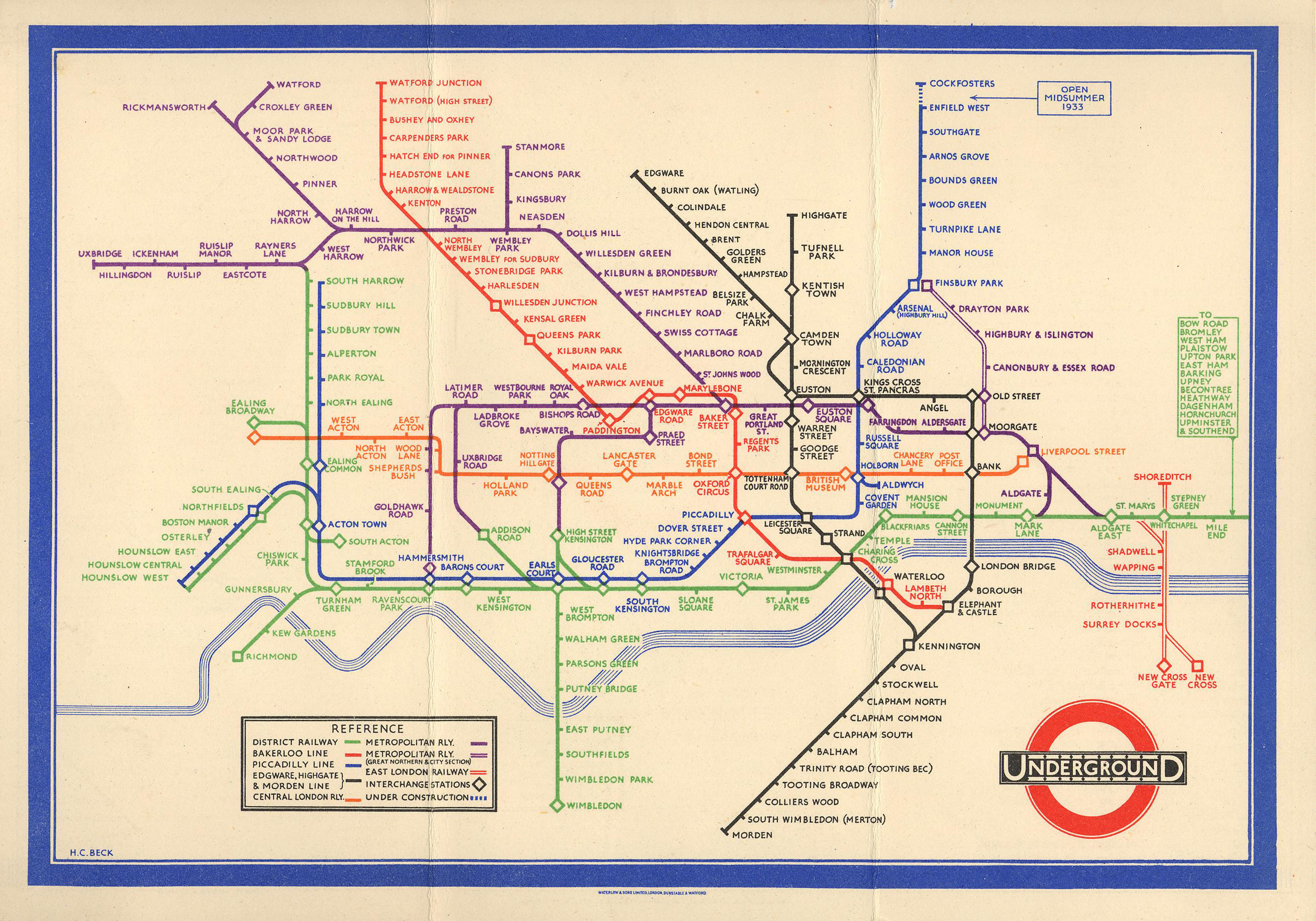You can see that the stations are vertices and the connecting tracks are the edges. This graph is not a digraph, because the edges do not have a direction. In reality edges may be traveled in both direction. You can trace with your pen or marker through the vertices and edges. Try it out and create a circuit (i.e. cycle) if you can.

Important property:  Important to notice that the map of 1933 is not accurate (not precise distances and proportions) when compared to the 2004 map, but it served the purpose equally well as the new and accurate map. It’s enough for passengers to know which station comes next and which line to use to get from A to B.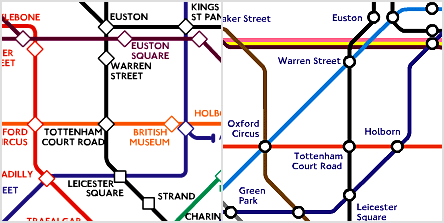Example 3: How about in the nature? Spider webs…Vertices are where the strands of web meet. Note that the edges do not have to be straight. Drops in the picture just let us see where the edges are and where the vertices they meet in are.

Masters of Magic (MOM)

screenshot with magic roads (and regular roads) – vertices and edges
486 dos game
Wholeness
Vertex-edge graphs consists of vertices (nodes i.e. dots) and edges (lines or arches) that allow us to represent and solve real-life problems graphically.

Example 5: Example vertices and edges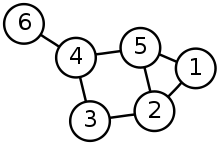A labeled graph with 6 vertices and 7 edges where the vertex number 6 on the far-left is a leaf vertex or a pendant vertex.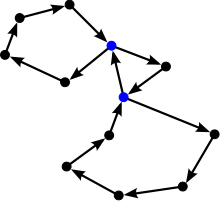A directed cycle. Without the arrows, it is just a cycle. This is not a simple cycle, since the blue vertices are used twice. This is an euler cycle, because all edges are used only once. This is not Hamiltonian cycle, because it uses vertices more than once.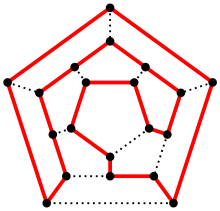This is a Hamiltonian path or cycle, because it goes through each vertex only once. It's also Euler cycle because it goes through each edge only once. It's a cycle because it ends where it starts.

Example 6: 2d to 3d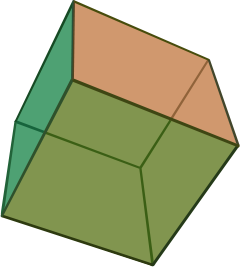Example 7: See how many vertices and edges you can find in this video? http://youtu.be/k-T7vGdH_ek

Exercises

See handout.

Homework